EXPERIMENT NO.6

 Time ( min) Content Learning Aid / Methodology Faculty Approach Typical Student Activity Skill / Competency Developed 10 Relevance and significance of experiment Chalk & Talk , Presentation Introduces, Facilitates, Monitors Listens, Participates, Discusses Knowledge, Communication, intrapersonal 20 Explanation of experiment Chalk & Talk , Presentation Introduces, Facilitates, Explains Listens Knowledge, Communication, intrapersonal, Application 60 Observations Role play/ demonstration Explains, Monitors Participates, Discusses Debate, comprehension, Hands on experiment 20 Calculations N/A Explains, Monitors Participates, Discusses Knowledge, Communication, Intrapersonal, Application 10 Results and conclusions Keywords Lists, Facilitates Listens, Participates, Discusses Knowledge, Communication, intrapersonal, Comprehension

TRANSMISSION LINE PARAMETERS.

Practical Session Plan

Title: Transmission line parameters.

Objectives: Set up the connections of transmission line to analyze the performance parameters

Aim:

Â· To explain the concept of transmission line

Â· To discuss concept of VSWR, impedance matching and effect of short and open circuit on these parameters

Apparatus: Transmission line Trainer kit.

Theory:

To analyze the behaviour of a transmission line connected between a generator and a load. For example in a transmitting antenna system, the transmitter is the generator and the antenna, the load in a receiving system; the antenna is the generator and the receiver, the load. Fig. shows a generator of voltage VG and internal impedance ZG connected to the load impedance ZL through a length d of a transmission line of characteristic impedance Z0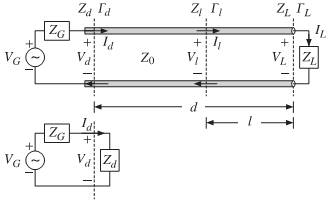Fig. Terminated lineâ€™s equivalent circuit.

Open- and short-circuited transmission lines are widely used to construct resonant circuits as well as matching stubs. They correspond to the special cases for the load impedance: ZL =âˆž for an open-circuited line and ZL = 0 for a short-circuited one. Fig. shows these two cases.Fig. Open- and short-circuited line and Thevenin -equivalent circuit.

Knowing the open-circuit voltage and the short-circuit current at the end terminals, b, allows us also to replace the entire left segment of the line, including the generator, with a Thevenin-equivalent circuit. Connected to a load impedance ZL, the equivalent circuit will produce the same load voltage and current VL, IL as the original line and generator. Setting ZL =âˆž and ZL = 0 in Eq. (10.9.2), we obtain the following expressions for the wave impedance Zl at distance l from the open- or short-circuited termination:

Zl =âˆ’jZ0 cot Î²l (open-circuited)

Zl = jZ0 tan Î²l (short-circuited)

The corresponding admittances Yl = 1/Zl will be:

Yl = jY0 tan Î²l (open-circuited)

Yl =âˆ’jY0 cot Î²l (short-circuited)

To determine the Thevenin -equivalent circuit that replaces everything to the left of the terminals a, b, we must ï¬nd the open-circuit voltage Vth, the short-circuit current Isc, and the Thevenin impedance Zth. The impedance Zth can be determined either by Z th = Vth/Isc, or by disconnecting the generator and finding the equivalent impedance looking to the left of the terminals a, b. It is obtained by propagating the generator impedance ZG by a distance d:

Zth = Z0(ZG + jZ0 tan Î² d )/(Z0 + jZG tan Î²d )

= Z0(1 + Î“th )/(1 âˆ’ Î“th )

where ,Î“th = Î“Geâˆ’2jÎ²d

The open-circuit voltage can be determined from equation by setting ZL =âˆž, which implies that Î“L = 1, Î“d = eâˆ’2jÎ²d, and Î“GÎ“d = Î“Ge âˆ’2jÎ²d= Î“th. The short-circuit current is also obtained from equation by setting ZL = 0, which gives Î“ L =âˆ’1,

Î“d =âˆ’eâˆ’2jÎ²d, and Î“GÎ“d =âˆ’Î“ Geâˆ’2jÎ²d=âˆ’Î“th.

Then, we find:

Vth =[ VGZ0 /(ZG + Z 0)][2eâˆ’jÎ²d/(1 âˆ’ Î“th)]

Isc = [VG /(ZG + Z0 )][(2eâˆ’jÎ²d)]/(1 + Î“th)]It follows that Vth/Isc = Zth, as given by Eq. A more convenient way of writing Eq. is by noting the relationships:

Hence,Where VÂ­, IÂ­ are the load voltage and currents in the matched case, given by Eq. The intuitive meaning of these expressions can be understood by writing them as:These are recognized to be the ordinary voltage and current dividers obtained by connecting the Thevenin and Norton equivalent circuits to the matched load impedance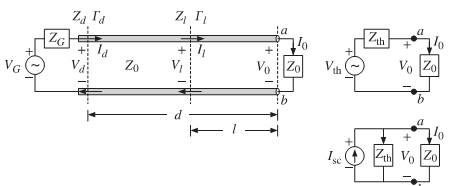Z0, as shown in Fig.

Fig.

Thevenin and Norton equivalent circuits connected to a matched load

The quantities V0, I0 are the same as those obtained by connecting the actual line to the matched load, as was done in Eq. An alternative way of determining the quantities Vth and Z th is by replacing the length-d transmission line segment by its T-section equivalent circuit, as shown in Fig.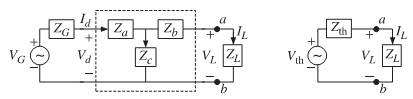Fig.T-section and Thevenin equivalent circuits

The Thevenin equivalent circuit to the left of the terminals a, b is easily determined by shorting the generator and finding the Thevenin impedance and then finding the open-circuit voltage. We have: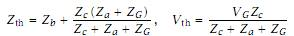where Za,Zb,Zc for a length-d segment are given by Eq.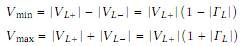Standing Wave Ratio: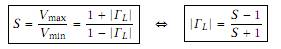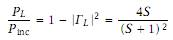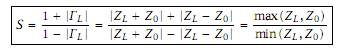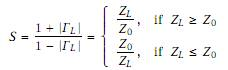Procedure:

1. Antenna trainer kit has four sections each of 25meter.Before doing any connection set generator voltage to 1 Volt/ 100 KHz. To measure accurate readings use DSO and keep it on measurement mode (Vpp& time).

2. Turn off kit supply and connect all sections to form continuous line of 100m.

3. To compromise source impedance, connect 18ohm impedance in series with source and transmission line.

4. For matched termination,

a) Total impedance of transmission line should be (50+18) ohms i.e.68 ohm. So terminate TL with impedance of this impedance for maximum power transfer.

b) Measure voltage Vpp at loadconsidering it as starting point of measurement (i.e load terminal at 0 meter). Draw the waveform observed.

c) Repeat same procedure for preceding nodes (i.e. at 25 meter, 50meter, 75meter & 100meter)

5. For Open and Short Termination,

a) Remove load of 68ohm from load terminal, measure voltage Vpp at this terminal as Voc at 0 meter; short this terminal and measure voltage Vpp at this terminal as Vsc at 0 meter. Draw the waveform observed.

b) Now remove the connection between 3rd and 4th block of transmission line. Measure voltage Vpp at this terminal as Voc at 25 meter; short this terminal and measure voltage V pp at this terminal as Vsc at25 meter. Draw the waveform observed.

c) Repeat same procedure for preceding nodes (i.e 50meter, 75meter & 100meter)

6. From observation table find maximum and minimum value of voltage measured and calculate VSWR, reflection coefficient using formulae given above.

Observations:

 Sr. No. Distance (m) For Matched For Open Circuit For Short Circuit termination termination termination 1. 100 2. 75 3. 50 4. 25 5. 0

Sample Calculations: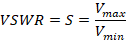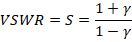Results:

 Sr.No. Matched termination Open Circuit termination Short Circuit termination VSWR Reflection Coeff.

Conclusion:

Value of reflection coefficient is minimum for matched termination as maximum power get dissipated into load whereas in open termination this value is maximum as all power reflects back to the source and in short termination value of reflection coefficient is same as open termination with negative sign since all power returns back with negative polarity.

Upon completion of experiment, student will be able to understand,

1. To understand the concept of transmission line.